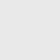# Capacitor Conversion

Welcome to our capacitance value conversion calculator, designed to help you effortlessly convert capacitance values between farads (F), picofarads (pF), and microfarads (μF). In addition to the conversion tool, our handy capacitor code conversion chart allows you to determine capacitance values by simply referencing the code.

Whether you are an electronics enthusiast, a student, or a professional working with capacitors, this tool will help you make quick and accurate conversions. Read on to learn how to use it effectively.

## How to Use the Calculator

2. Input Value: Enter the capacitance value you wish to convert in the provided input field. The calculator accommodates a broad range of capacitance values, from nano to micro to farads.
3. Get Result: Once you've entered your value, simply click the 'Calculate' button. Instantly, the converted capacitance value in your chosen unit will be displayed clearly on the screen.

Also, Check out our amazing Resistor color code calculator

## Common Capacitance Conversions

Here are some common capacitance conversions you might find useful with this calculator:

• Farads to Picofarads (F to pF): Convert large capacitance values into smaller units. For example, 1 F is equivalent to 1,000,000,000 pF.
• Farads to Microfarads (F to μF): Transform large capacitance values into more practical values. 1 F equals 1,000,000 μF.
• Picofarads to Farads (pF to F): For precise calculations, convert small capacitance values into farads. 1 pF is equal to 1e-12 F.
• Picofarads to Microfarads (pF to μF): Convert small values into practical units. 1 pF is equal to 0.000001 μF.
• Microfarads to Farads (μF to F): Use this conversion when dealing with practical capacitance values. 1 μF is equivalent to 0.000001 F.

## Subscribe to our weekly newsletter

Subscribe
Notify ofInline Feedbacks

## Browse## Browse